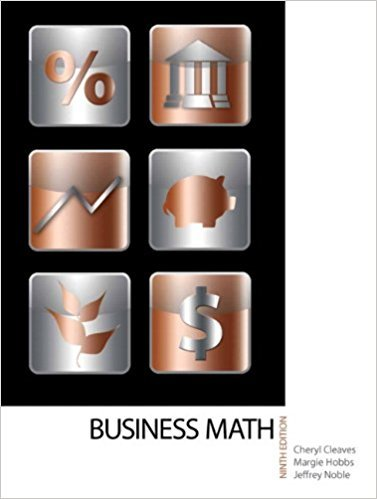×
×

# Solutions for Chapter 6-3: INCREASES AND DECREASES## Full solutions for Business Math, | 9th Edition

ISBN: 9780135108178Solutions for Chapter 6-3: INCREASES AND DECREASES

Solutions for Chapter 6-3
4 5 0 307 Reviews
16
2
##### ISBN: 9780135108178

Since 18 problems in chapter 6-3: INCREASES AND DECREASES have been answered, more than 17376 students have viewed full step-by-step solutions from this chapter. Business Math, was written by and is associated to the ISBN: 9780135108178. This expansive textbook survival guide covers the following chapters and their solutions. This textbook survival guide was created for the textbook: Business Math, , edition: 9. Chapter 6-3: INCREASES AND DECREASES includes 18 full step-by-step solutions.

Key Math Terms and definitions covered in this textbook
• Basis for V.

Independent vectors VI, ... , v d whose linear combinations give each vector in V as v = CIVI + ... + CdVd. V has many bases, each basis gives unique c's. A vector space has many bases!

• Change of basis matrix M.

The old basis vectors v j are combinations L mij Wi of the new basis vectors. The coordinates of CI VI + ... + cnvn = dl wI + ... + dn Wn are related by d = M c. (For n = 2 set VI = mll WI +m21 W2, V2 = m12WI +m22w2.)

A sequence of steps (end of Chapter 9) to solve positive definite Ax = b by minimizing !x T Ax - x Tb over growing Krylov subspaces.

• Cross product u xv in R3:

Vector perpendicular to u and v, length Ilullllvlll sin el = area of parallelogram, u x v = "determinant" of [i j k; UI U2 U3; VI V2 V3].

• Ellipse (or ellipsoid) x T Ax = 1.

A must be positive definite; the axes of the ellipse are eigenvectors of A, with lengths 1/.JI. (For IIx II = 1 the vectors y = Ax lie on the ellipse IIA-1 yll2 = Y T(AAT)-1 Y = 1 displayed by eigshow; axis lengths ad

• Factorization

A = L U. If elimination takes A to U without row exchanges, then the lower triangular L with multipliers eij (and eii = 1) brings U back to A.

• Graph G.

Set of n nodes connected pairwise by m edges. A complete graph has all n(n - 1)/2 edges between nodes. A tree has only n - 1 edges and no closed loops.

• Hermitian matrix A H = AT = A.

Complex analog a j i = aU of a symmetric matrix.

• Hessenberg matrix H.

Triangular matrix with one extra nonzero adjacent diagonal.

• Iterative method.

A sequence of steps intended to approach the desired solution.

• Krylov subspace Kj(A, b).

The subspace spanned by b, Ab, ... , Aj-Ib. Numerical methods approximate A -I b by x j with residual b - Ax j in this subspace. A good basis for K j requires only multiplication by A at each step.

• lA-II = l/lAI and IATI = IAI.

The big formula for det(A) has a sum of n! terms, the cofactor formula uses determinants of size n - 1, volume of box = I det( A) I.

• Linear transformation T.

Each vector V in the input space transforms to T (v) in the output space, and linearity requires T(cv + dw) = c T(v) + d T(w). Examples: Matrix multiplication A v, differentiation and integration in function space.

• Partial pivoting.

In each column, choose the largest available pivot to control roundoff; all multipliers have leij I < 1. See condition number.

• Positive definite matrix A.

Symmetric matrix with positive eigenvalues and positive pivots. Definition: x T Ax > 0 unless x = O. Then A = LDLT with diag(D» O.

• Random matrix rand(n) or randn(n).

MATLAB creates a matrix with random entries, uniformly distributed on [0 1] for rand and standard normal distribution for randn.

• Skew-symmetric matrix K.

The transpose is -K, since Kij = -Kji. Eigenvalues are pure imaginary, eigenvectors are orthogonal, eKt is an orthogonal matrix.

• Solvable system Ax = b.

The right side b is in the column space of A.

• Symmetric matrix A.

The transpose is AT = A, and aU = a ji. A-I is also symmetric.

• Vector v in Rn.

Sequence of n real numbers v = (VI, ... , Vn) = point in Rn.

×